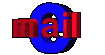Earth and Space Systems
The force of gravity found between the Earth and the Moon is the cause of the ocean tides. Before we get into tides, we should take a look at this force called gravity.
Gravity:
We know that the earth rotates or spins but why doesn't everything go flying off? Why don't we just fall off of the earth and go floating up into space? The Answer: GRAVITY. We stick to the
earth. Think of it as being held onto the ground. Gravity is a force between things (objects) that causes the objects to be attracted to one another. Sir Isaac Newton, a scientist from the mid-1600, realized that the force which kept the moon in its orbit was the same as the force which caused an apple to fall to the ground. He eventually developed a theory which became a law and is known as the Law of Gravity. All objects exert a force of gravity. How strong the gravity will be depends on two things: a) the size of the objects and b) the distance between them.
Now, lets talk about mass and weight. Think of mass as the amount of stuff. Here's a good example. Your math text book is made up of paper, ink etc. Its mass will stay the same whether you pick up the book today, tomorrow, put it under your bed or in your closet. The mass of the text book does not change.
Weight is a bit different. Weight is the amount of force that is being pushed onto the ground. Take your math text book as an example. How much force is pushing it onto the chair, table or floor? This force is the weight; it is also the gravity. So, if we take your math book on a trip to the moon, the mass of your book will be the same but the weight of the book will be different because the force of gravity is different. In space, your math text book will float around but it still has the same pages and ink, the mass doesn't change.
By the way, a fact of interest for you. The moon's gravity is 1/6 as strong as earth's. That means that if you weigh 66 kg ( about 145 lbs ) and you went to the moon, your weight would be 1/6. You would only weigh 11 kg ( about 24 lbs ).
Another fact of interest! Gravity on earth isn't the same everywhere. The gravity is less at the equator then it is at the two poles. That means you would weigh less at the equator then you would at the North Pole.
Ok now, back to tides.
Tides:
As I mentioned, the gravitational force between the earth and moon is what causes our tides. This invisible force pulls at the earth's water. As the moon and earth orbit the sun their positions or locations are constantly shifting. This causes different gravitational effects which in turn cause the tides to not only vary from place to place but also the times of the tides will be different. The gravitational force causes the water to bulge out. When the sun, moon and earth are all lined up, at new and full moon, see phases of moon then the bulge is much larger creating higher tides than normal. These tides are called Spring Tides. When the sun is at a right angle or 90   the sun and moon work against each other so the tides are very low. These are called Neap Tides. As the earth rotates the water bulges near the moon - high tide. When the moon is at a 90   angle to the earth then there will be low tides.
o
omoon
earth
high tide
high tide
low tide
low tide